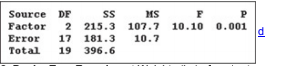×
Get Full Access to Elementary Statistics - 12 Edition - Chapter 12.3 - Problem 1 re
Get Full Access to Elementary Statistics - 12 Edition - Chapter 12.3 - Problem 1 re

×

Solved: Car Weight and Fuel Consumption Data Set 14 inISBN: 9780321836960 18

Solution for problem 1 RE Chapter 12.3

Elementary Statistics | 12th Edition

• Textbook Solutions
• 2901 Step-by-step solutions solved by professors and subject experts
• Get 24/7 help from StudySoup virtual teaching assistantsElementary Statistics | 12th Edition

4 5 1 243 Reviews
30
5
Problem 1 RE

Car Weight and Fuel Consumption Data Set 14 in Appendix B includes highway fuel consumption amounts for cars with 4 cylinders, 6 cylinders, and 8 cylinders. (The 5­cylinder Volvo S60 is excluded from this exercise.) Analysis of variance is used with the highway fuel consumption amounts (mi/gal) categorized according to the number of cylinders (4, 6, 8); the results are shown in the following display. Identify the null hypothesis, test statistic, and P­-value, and state the final conlusion in nontechnical terms.

MINITABStep-by-Step Solution:
Step 1 of 3

Solution  1RE

It is seen that, value of test statistic is 10.1 .

In this case, one-way analysis of variance is one tailed test, so the P-value corresponding to the F statistic is 0.001 .

In this case, one-way analysis of variance is one tailed test, so the P-value corresponding to the F statistic is 0.001 . Comparing the P-value with the significance level 0.05 , it is seen that the P-value is less than the significance level 0.05 . So, the null hypothesis is rejected.

Step 2 of 3

Step 3 of 3

ISBN: 9780321836960

Since the solution to 1 RE from 12.3 chapter was answered, more than 280 students have viewed the full step-by-step answer. This full solution covers the following key subjects: cylinders, consumption, Fuel, highway, amounts. This expansive textbook survival guide covers 121 chapters, and 3629 solutions. Elementary Statistics was written by and is associated to the ISBN: 9780321836960. This textbook survival guide was created for the textbook: Elementary Statistics, edition: 12. The answer to “?Car Weight and Fuel Consumption Data Set 14 in Appendix B includes highway fuel consumption amounts for cars with 4 cylinders, 6 cylinders, and 8 cylinders. (The 5­cylinder Volvo S60 is excluded from this exercise.) Analysis of variance is used with the highway fuel consumption amounts (mi/gal) categorized according to the number of cylinders (4, 6, 8); the results are shown in the following display. Identify the null hypothesis, test statistic, and P­-value, and state the final conlusion in nontechnical terms.MINITAB” is broken down into a number of easy to follow steps, and 81 words. The full step-by-step solution to problem: 1 RE from chapter: 12.3 was answered by , our top Statistics solution expert on 03/15/17, 10:30PM.

Unlock Textbook Solution